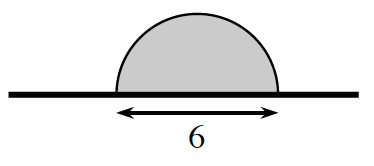Home > APCALC > Chapter 1 > Lesson 1.2.1 > Problem1-23

1-23.

A semi-circular flag is shown attached to a “pole” at right.1. Imagine rotating the flag about its pole and describe the resulting three-dimensional figure. Draw a picture of this figure on your paper.

You can build a model of a rotating flag problem. All you need is a pencil, scissors, tape and some paper. To build your model, cut out a flag and tape it to a pencil (which represents the flagpole). Once assembled, spin the pencil rapidly between your hands. If you spin the pencil fast enough, you will see a 3D figure. In this case, it will be a sphere.

1. Calculate the volume of the rotated flag.

$\text{volume of a sphere}=\frac{4}{3}\pi r^3$

Be careful! The radius of this sphere is not $6$.

Use the eTool below to visualize the flag.
Click the link at right for the full version of the eTool: Calc 1-23 HW eTool